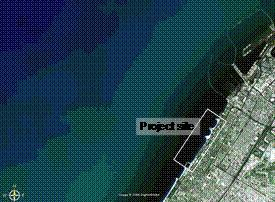# Process-based morphological models

The so-called area models, which combine waves, currents and sediment transport models and include updating of the model bathymetries, have proven to be very strong tools in the short to medium term (days to weeks) for the study of morphological impacts from significant changes to the coastal system. The present article discusses how such models can be applied for longer time frames.

## The Morphological Area Models

The two dimensional so-called area models consist of a series of modules capable of simulating different wave-related, current and sediment transport processes. The three models are combined into a morphological modelling system, see for instance Johnson et al (1994) , Johnson and Zyserman (2002)  and Niemann (2006) . The modules are combined as shown in Fig. 1.

The morphological area models can be set up for areas of the order of magnitude 10 by 10 km and be operated realistically for about 2 to 3 months real time. The resolution has to be fine, down to approx. 5-10 m in the surf zone.

Two ways of utilising this type of models in the most efficient way are discussed in the sections “Input Schematisation” and “Speed-up of the Sediment Transport Rates”. Furthermore, the use of the model complex to calculate an “equilibrium” bathymetry is discussed.

## Input Schematisation

This method is illustrated by a case which is discussed in more detail in Johnson et al (2005). The concept of this method is to simulate the events, which contribute significantly to the morphological development only. The project site is shown in Fig. 2. It is a sandy, moderately exposed coast. The example focuses on sedimentation behind a shore parallel breakwater. Fig. 3 shows the bathymetries as measured in March 1995 and in January 1997, i.e. a period which is too long for morphological modelling within a reasonable time frame.Fig. 2. Project site, for illustration of input schematisation.

The dominant driving forces are the waves. It has therefore been chosen to construct a time series of wave parameters along the offshore boundary of the model area which consists of situations where the significant wave height exceeds 1.5 m only. The compressed time series has a length of 42 days which can be compared to the original 21 months. The input schematisation is illustrated in Figure 4, which shows the first 3 months of the original time series and the compressed time series.

The full morphological modelling complex is used to simulate the compressed time series. The simulated morphological development is compared to the observed evolution. Various model parameters were fine tuned for optimal calibration. Results from the final calibration are shown in Fig. 5. After calibration, the modelling complex was applied to test various schemes.

## Speed–up of the Sediment Transport Rates

“Speed-up” techniques can be used in different ways. The simplest way is to multiply the calculated sediment transport with a speed-up factor and in this way make the simulation represent more than the physically modelled time period. This method is useful in cases where wave driven currents are the dominant phenomena and for instance in cases where the simulation is used to find a new equilibrium depth, see below example. A more complex method is to calculate the average sediment transport field for a given period of time, a tidal cycle, or a neap-spring period, derive the average bed level change rates and use this rate for updating of the bathymetry. The average bed level change rates can then be multiplied by a speed-up factor. Such a method requires that the time scale of significant morphological changes are long compared to the period of time for which average bed level change rates are calculated.

Careful calibration and validation are required in all cases where speed-up techniques are applied. The simple speed-up technique is illustrated in the example presented in Fig. 6. The upper figure shows a one-month long time series of offshore waves, height and direction. The middle figure shows the morphological evolution in the vicinity of a planned harbour during the one month (contours are the initial bathymetry whereas colours are depths after the one month). The lower figure shows the time series of bed level changes in 4 positions off the entrance. It appears that the morphological changes are small, less than 20 cm in the most active parts of the area. Fig. 7 shows the result of the simulation of the same period of time now with a speed-up factor of 25 on the sediment transport rates. Now the calculated bed level changes are clearly seen and even the tendency for the formation of a stronger rip channel is apparent. This gain in physical time has increased the number of morphological time steps. However, in the present case the increase is about 20%.Fig.7. Morphological changes for the same month as shown in Figure 6, but with a speed-up factor of 25 on the sediment transport.

## “Equilibrium” Morphology

The morphological models have proven very useful to predict the changes in the morphology due to major changes such as construction of groynes, breakwaters, revetments or harbours in the coastal zone. One way of using the models is to study the bathymetry which will develop under constant wave action after a long time period. Fig. 8 shows the result of morphological modelling of the bathymetry around the harbour shown in Figs. 6 and 7. The driving force is now constant waves from south east. The wave driven currents will concentrate around the harbour and with time an equilibrium depth will develop which allows the amount of sand fed in from the up-drift side to bypass the harbour. In the present case it appears after some modifications that an equilibrium depth of approximately 8 m will develop in front of the present harbour.